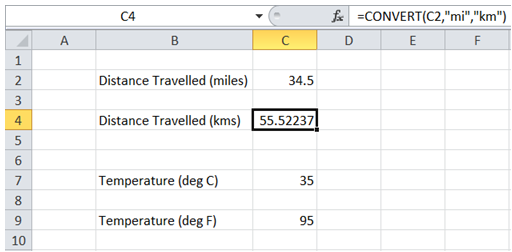## Convert measurement units in Excel

For example, I want to convert 34.5 miles in my Excel worksheet to kms or 35 deg C to Fahrenheit.

To do it in Excel, here is the answer:

a) Enter the formula =CONVERT(C2,"mi","km") where C2 corresponds to distance data in "Miles".b) Similarly, enter the formula =CONVERT(C7,"C","F") where C7 corresponds to Temperature reading in "Degrees Celsius".